Type
Quiz
Book Title
Vector Mechanics for Engineers: Statics and Dynamics 11th Edition
ISBN 13
978-0073398242

### 978-0073398242 Chapter 13 Solution Manual Part 7

February 25, 2020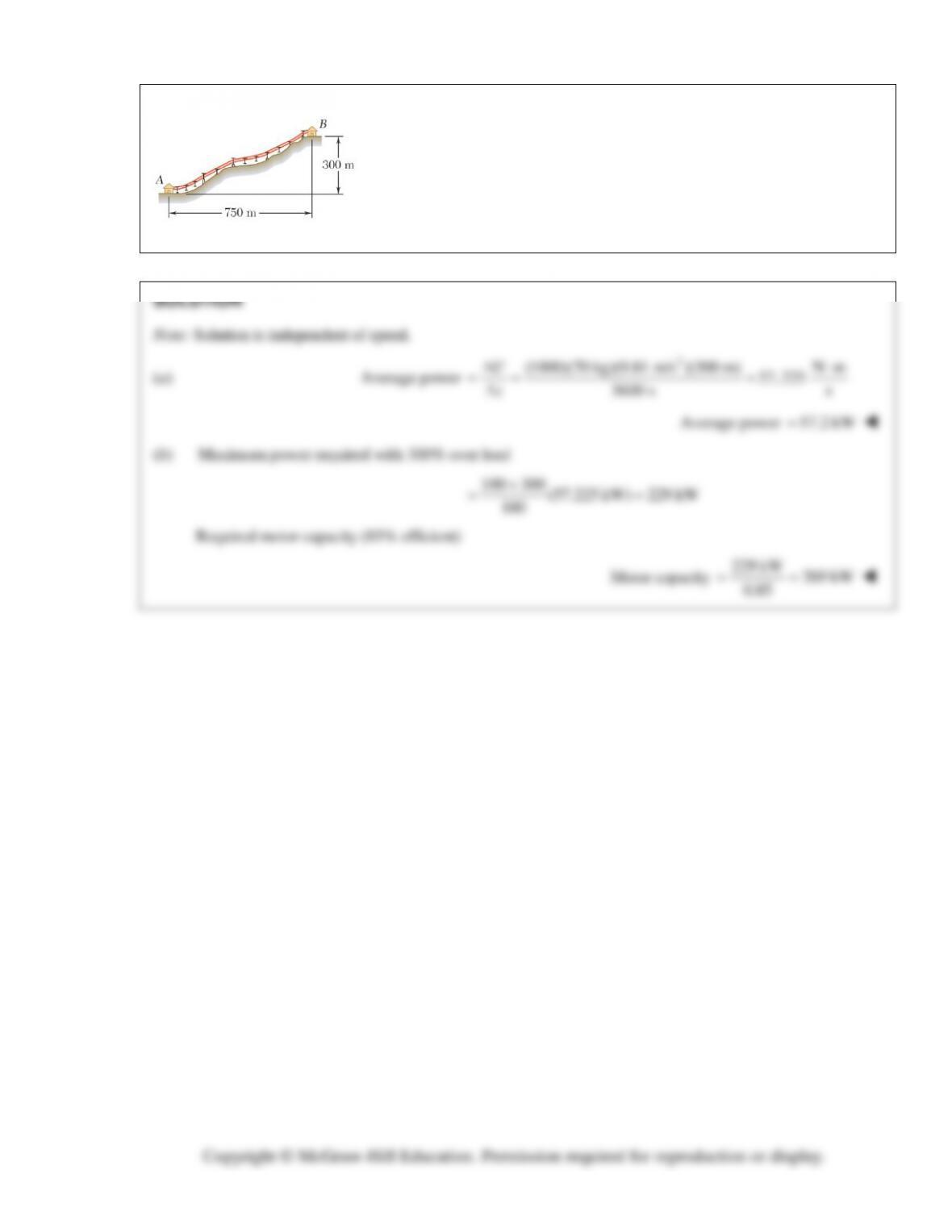PROBLEM 13.46
A chair-lift is designed to transport 1000 skiers per hour from the base A to
the summit B. The average mass of a skier is 70 kg and the average speed of
the lift is 75 m/min. Determine (a) the average power required, (b) the
required capacity of the motor if the mechanical efficiency is 85 percent and
if a 300 percent overload is to be allowed.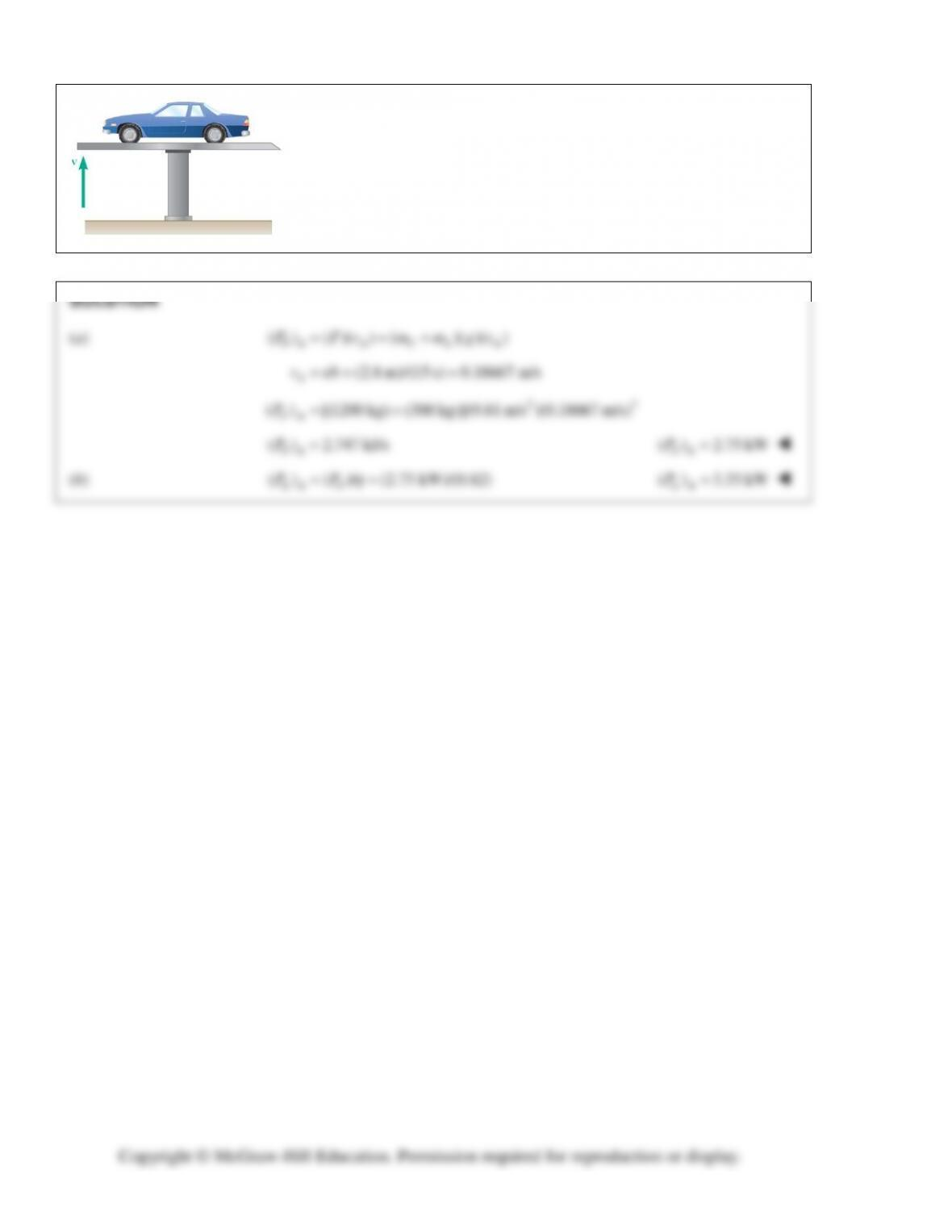PROBLEM 13.47
It takes 15 s to raise a 1200-kg car and the supporting 300-kg hydraulic
car-lift platform to a height of 2.8 m. Determine (a) the average output
power delivered by the hydraulic pump to lift the system, (b) the average
power electric required, knowing that the overall conversion efficiency
from electric to mechanical power for the system is 82 percent.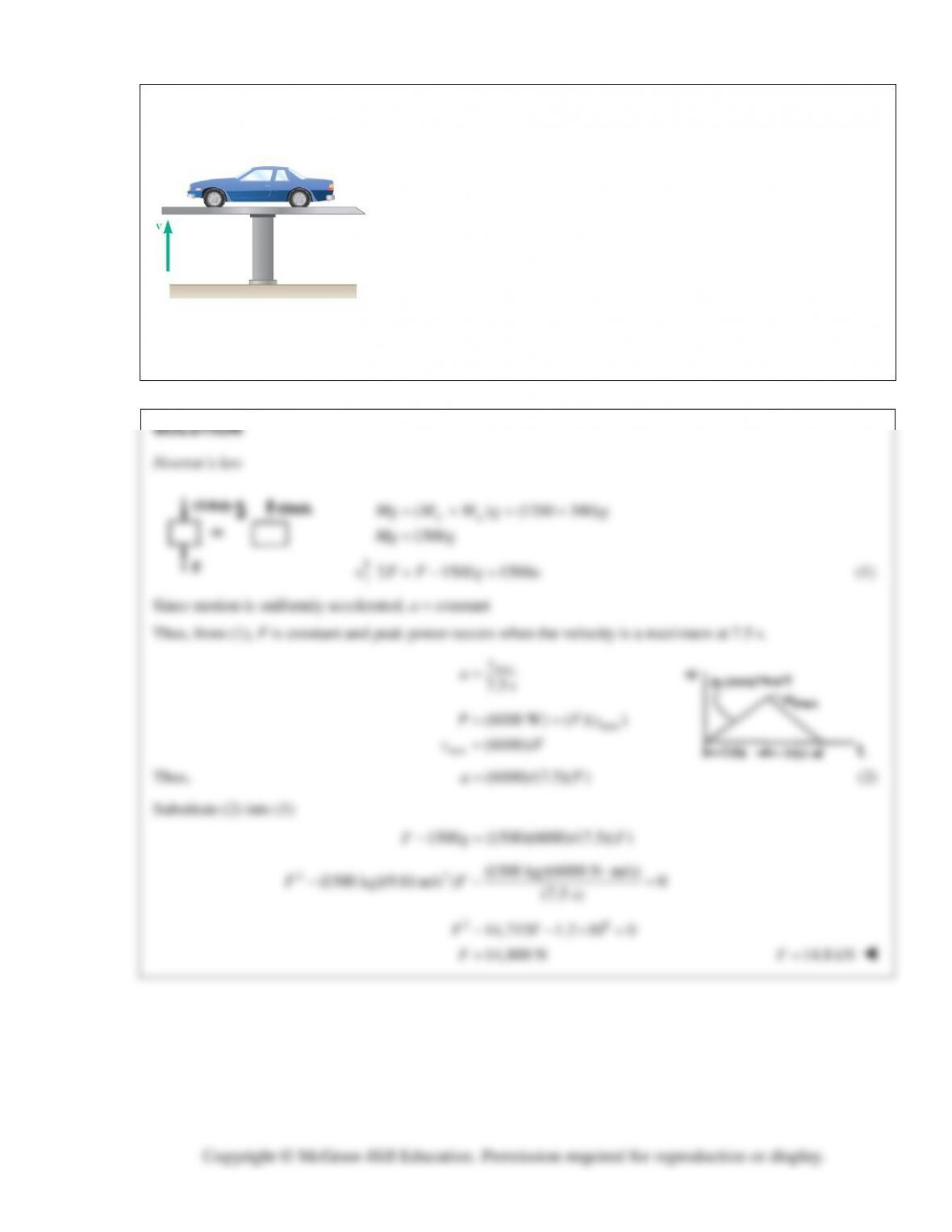PROBLEM 13.48
The velocity of the lift of Problem 13.47 increases uniformly from zero to
its maximum value at mid-height 7.5 s and then decreases uniformly to
zero in 7.5 s. Knowing that the peak power output of the hydraulic pump is
6 kW when the velocity is maximum, determine the maximum life force
provided by the pump.
PROBLEM 13.47 It takes 15 s to raise a 1200-kg car and the supporting
300-kg hydraulic car-lift platform to a height of 2.8 m. Determine
(a) the average output power delivered by the hydraulic pump to lift the
system, (b) the average power electric required, knowing that the overall
conversion efficiency from electric to mechanical power for the system is
82 percent.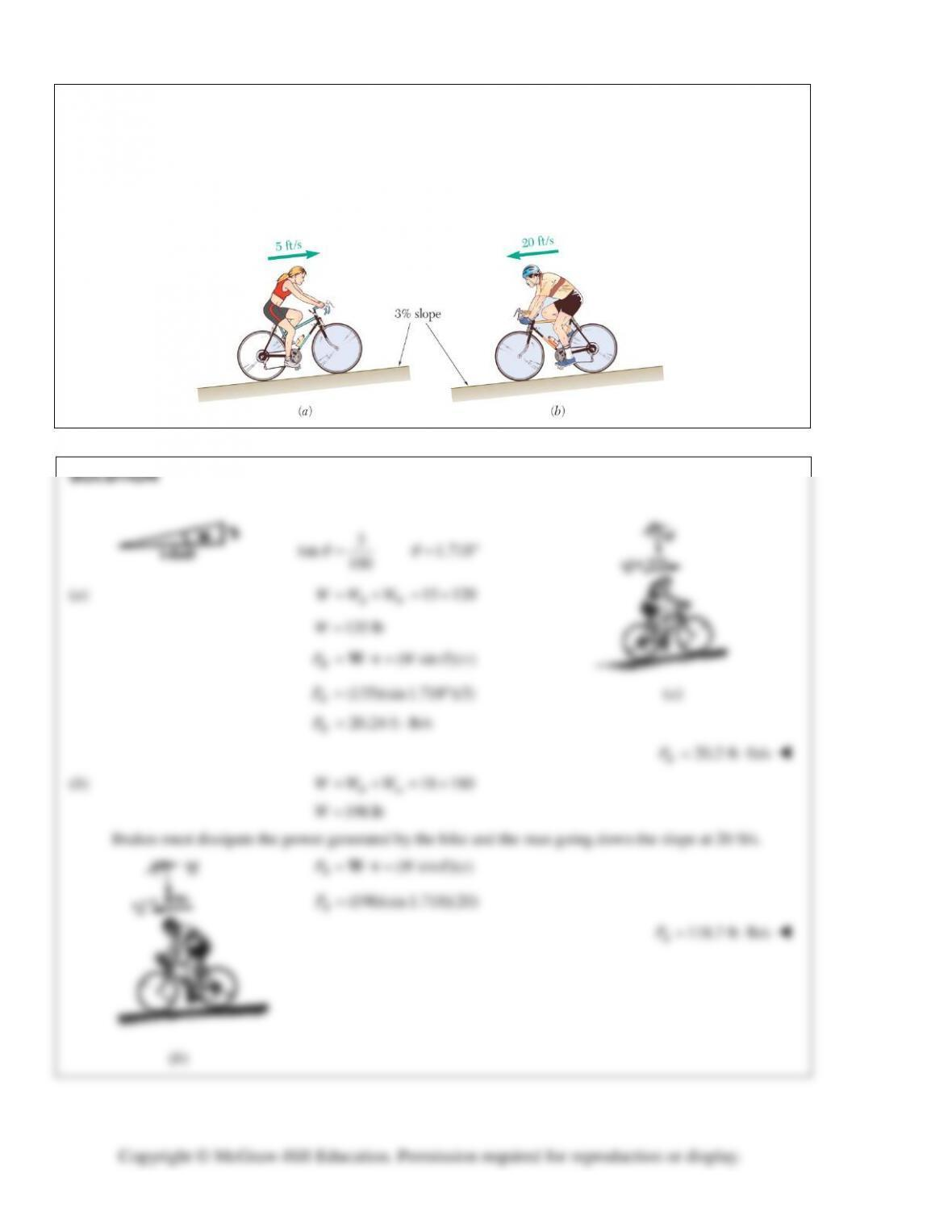PROBLEM 13.49
(a) A 120-lb woman rides a 15-lb bicycle up a 3-percent slope at a constant speed of 5 ft/s. How much power
must be developed by the woman? (b) A 180-lb man on an 18-lb bicycle starts down the same slope and
maintains a constant speed of 20 ft/s by braking. How much power is dissipated by the brakes? Ignore air
resistance and rolling resistance.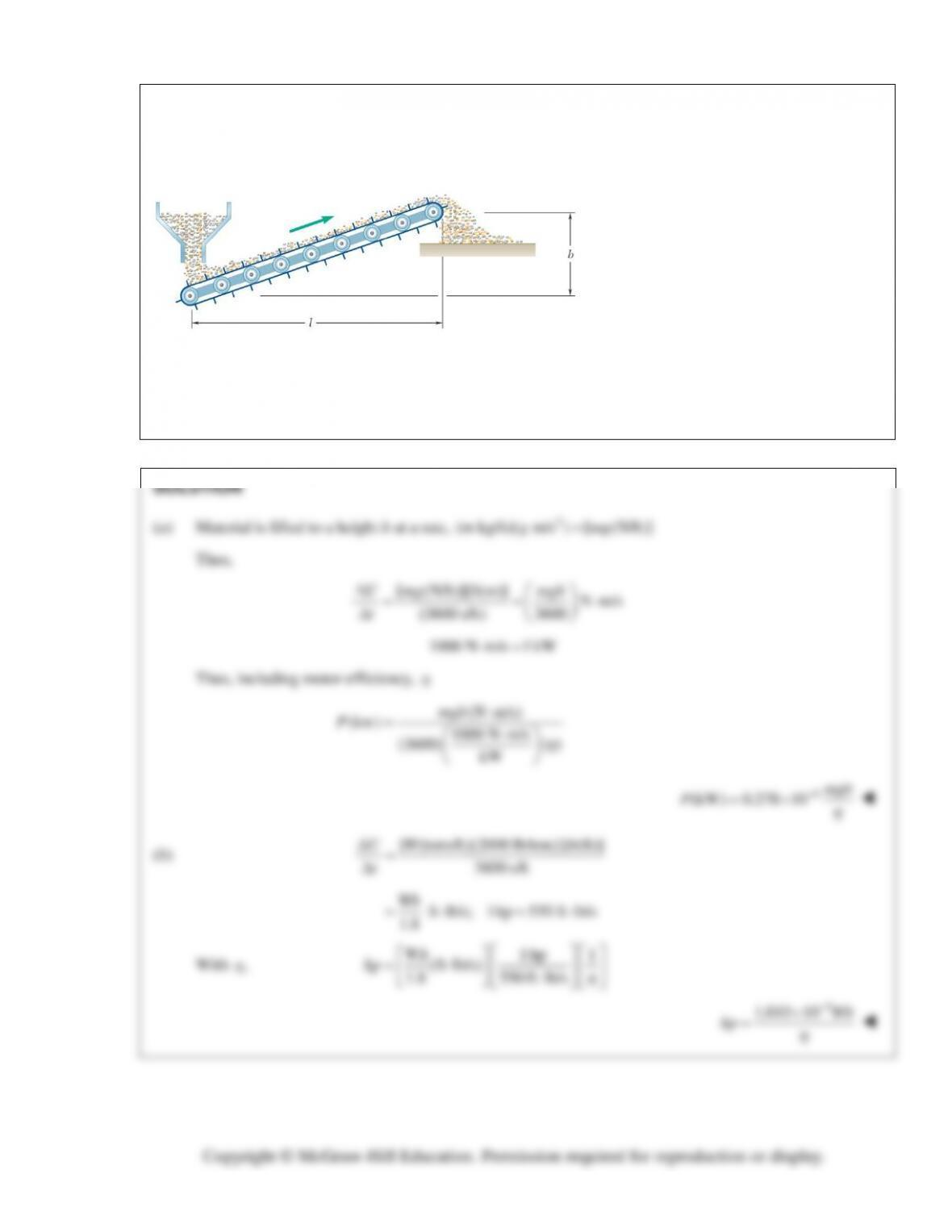PROBLEM 13.50
A power specification formula is to be
derived for electric motors which drive
conveyor belts moving solid material at
different rates to different heights and
distances. Denoting the efficiency of the
motors by
η
and neglecting the power
needed to drive the belt itself, derive a
formula (a) in the SI system of units for
the power P in kW, in terms of the mass
flow rate m in kg/h, the height b and
horizontal distance l in meters, and (b) in
U.S. customary units, for the power in hp,
in terms of the material flow rate
w
in
tons/h, and the height b and horizontal
distance l in feet.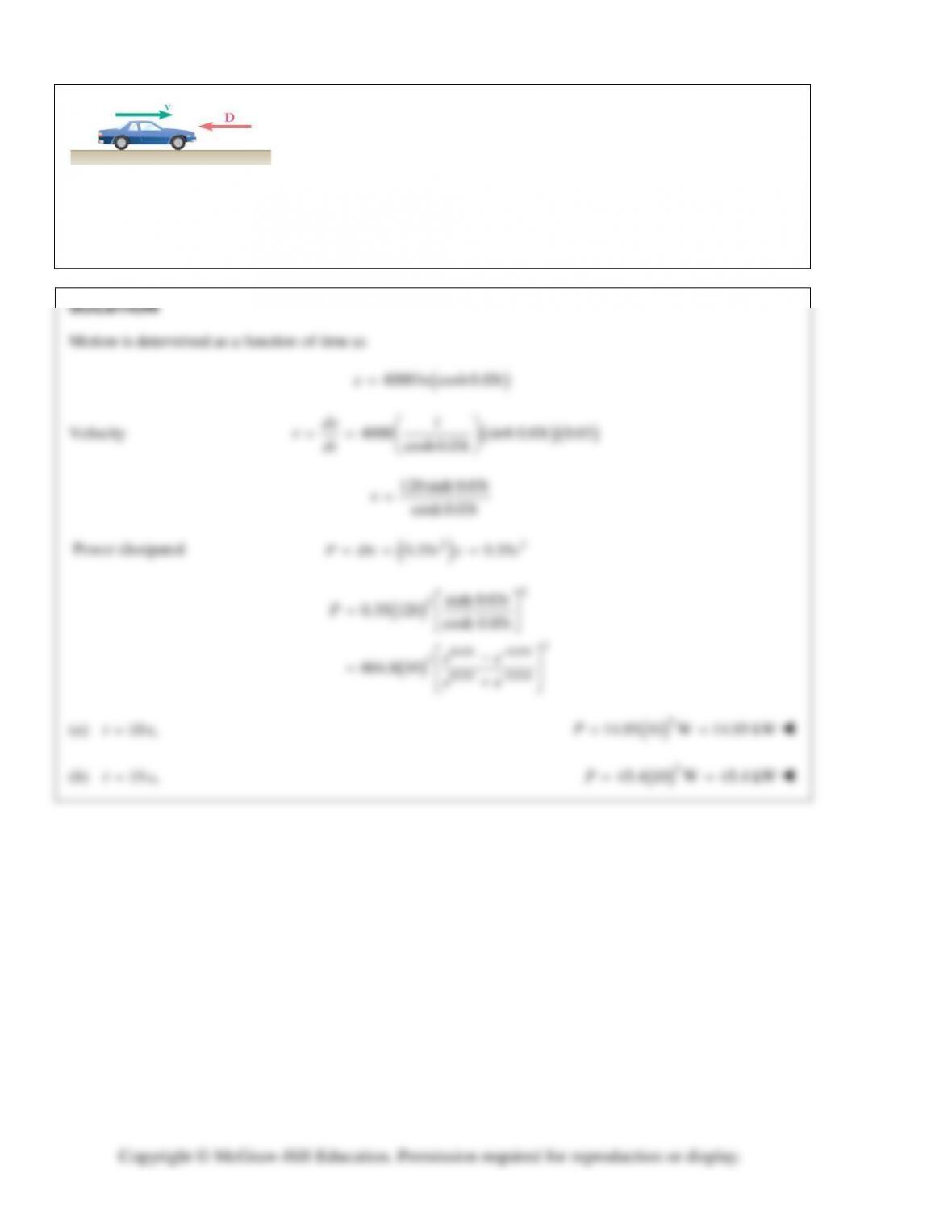PROBLEM 13.51
A 1400-kg automobile starts from rest and travels 400 m during a
performance test. The motion of the automobile is defined by the relation
4000ln(cosh0.03 ),xt=
where x and t are expressed in meters and seconds,
respectively. The magnitude of the aerodynamic drag is
2
0.35 ,Dv
=
where
D and
v
are expressed in newtons and m/s, respectively. Determine the
power dissipated by the aerodynamic drag when (a) t = 10 s, (b) t = 15 s.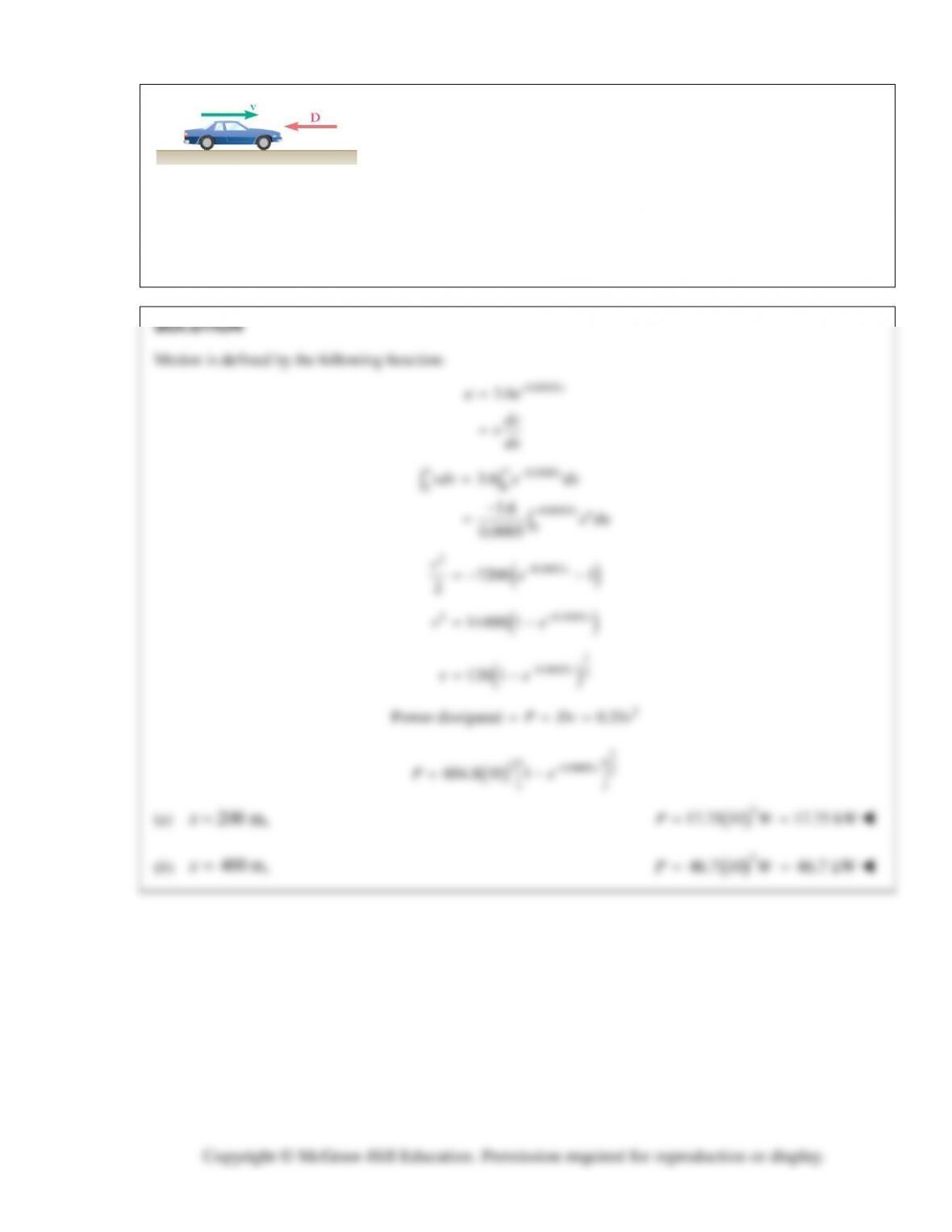PROBLEM 13.52
A 1400-kg automobile starts from rest and travels 400 m during a
performance test. The motion of the automobile is defined by the relation
0.0005
3.6
x
ae
=
, where a and x are expressed in
2
m/s
and meters,
respectively. The magnitude of the aerodynamic drag is
2
0.35 ,Dv=
where D and
v
are expressed in newtons and m/s, respectively. Determine
the power dissipated by the aerodynamic drag when (a) x = 200 m,
(b) x = 400 m.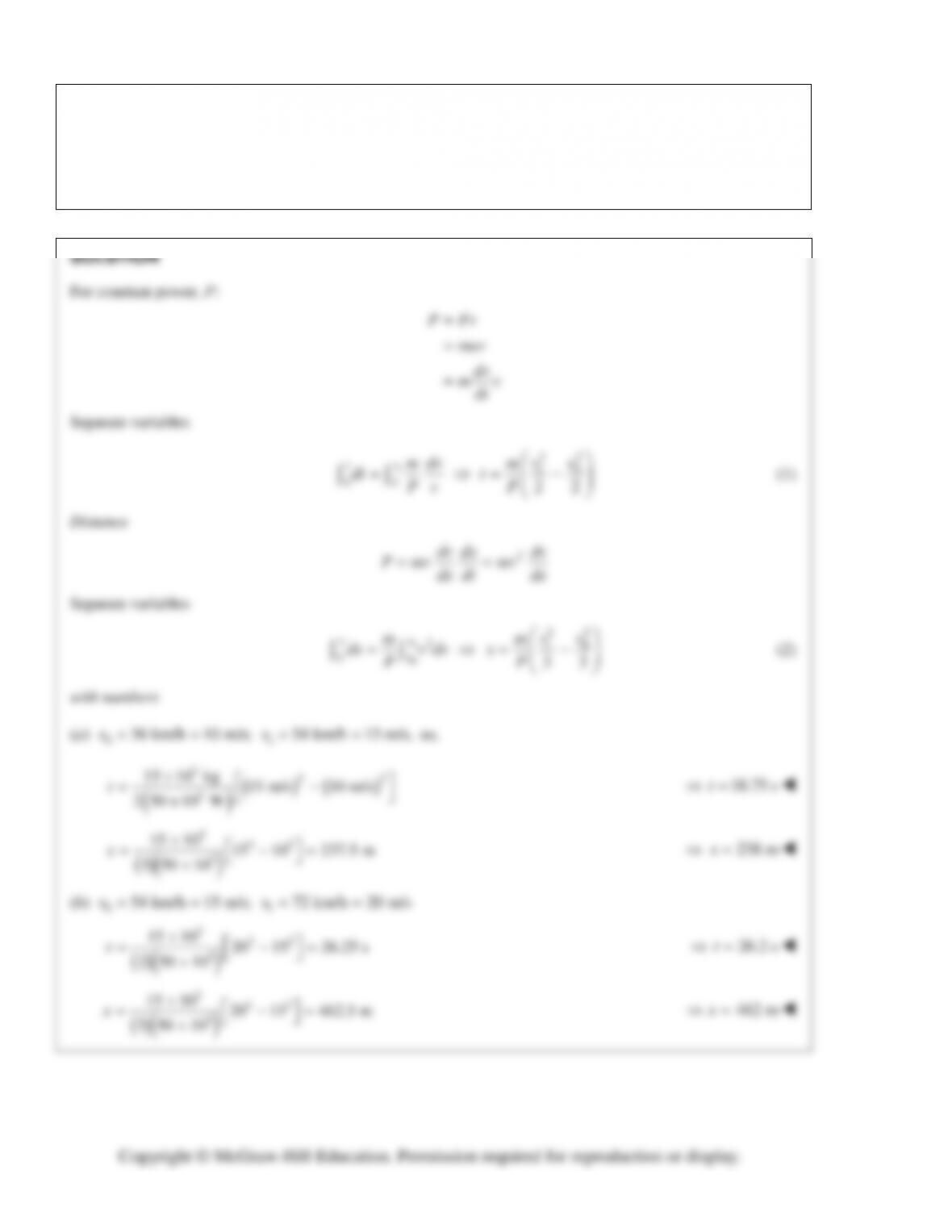PROBLEM 13.53
The fluid transmission of a 15-Mg truck allows the engine to deliver an essentially constant power of 50 kW
to the driving wheels. Determine the time required and the distance traveled as the speed of the truck is
increased (a) from 36 km/h to 54 km/h, (b) from 54 km/h to 72 km/h.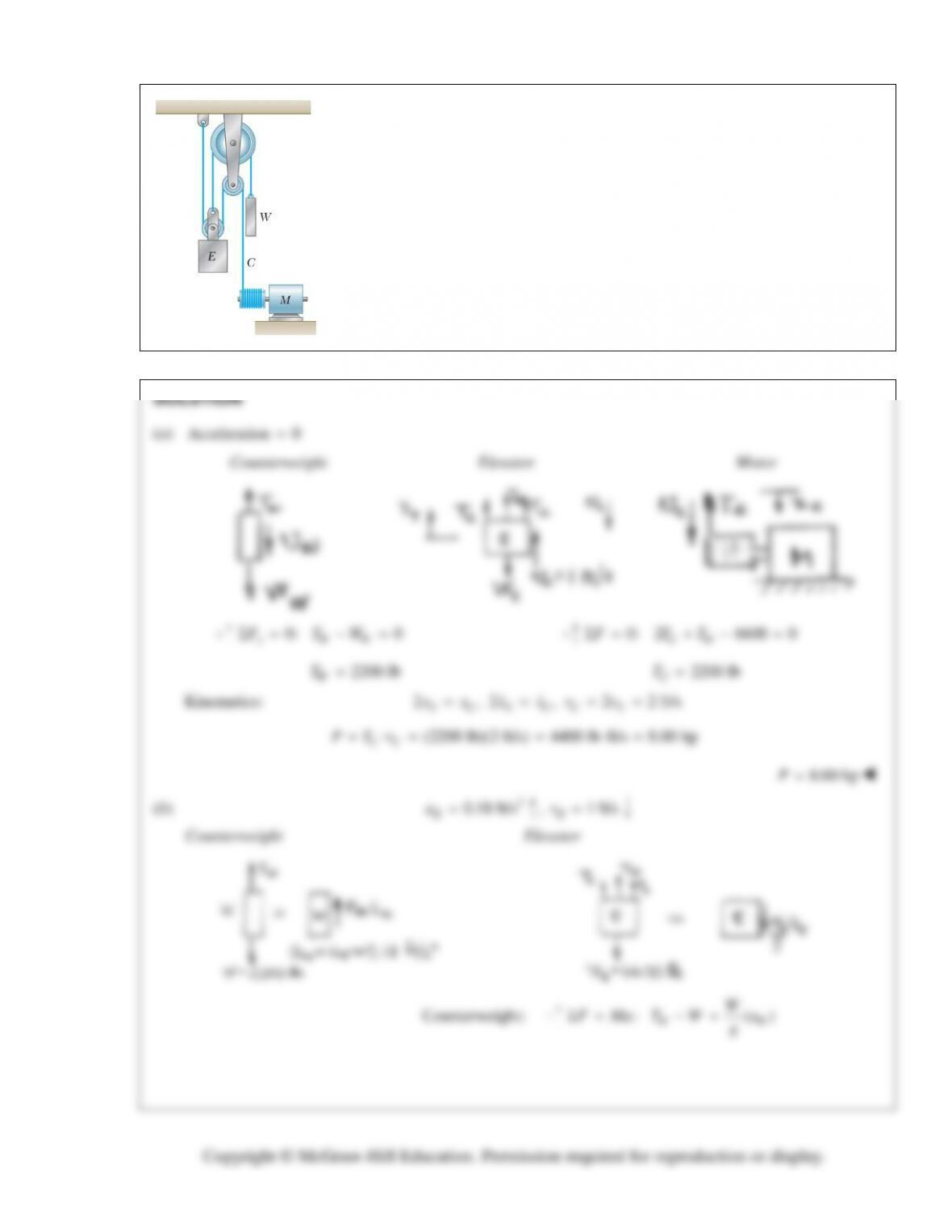PROBLEM 13.54
The elevator E has a weight of 6600 lbs when fully loaded and is connected as
shown to a counterweight W of weight of 2200 lb. Determine the power in hp
delivered by the motor (a) when the elevator is moving down at a constant speed
of 1 ft/s, (b) when it has an upward velocity of 1 ft/s and a deceleration of
2
0.18 ft/s .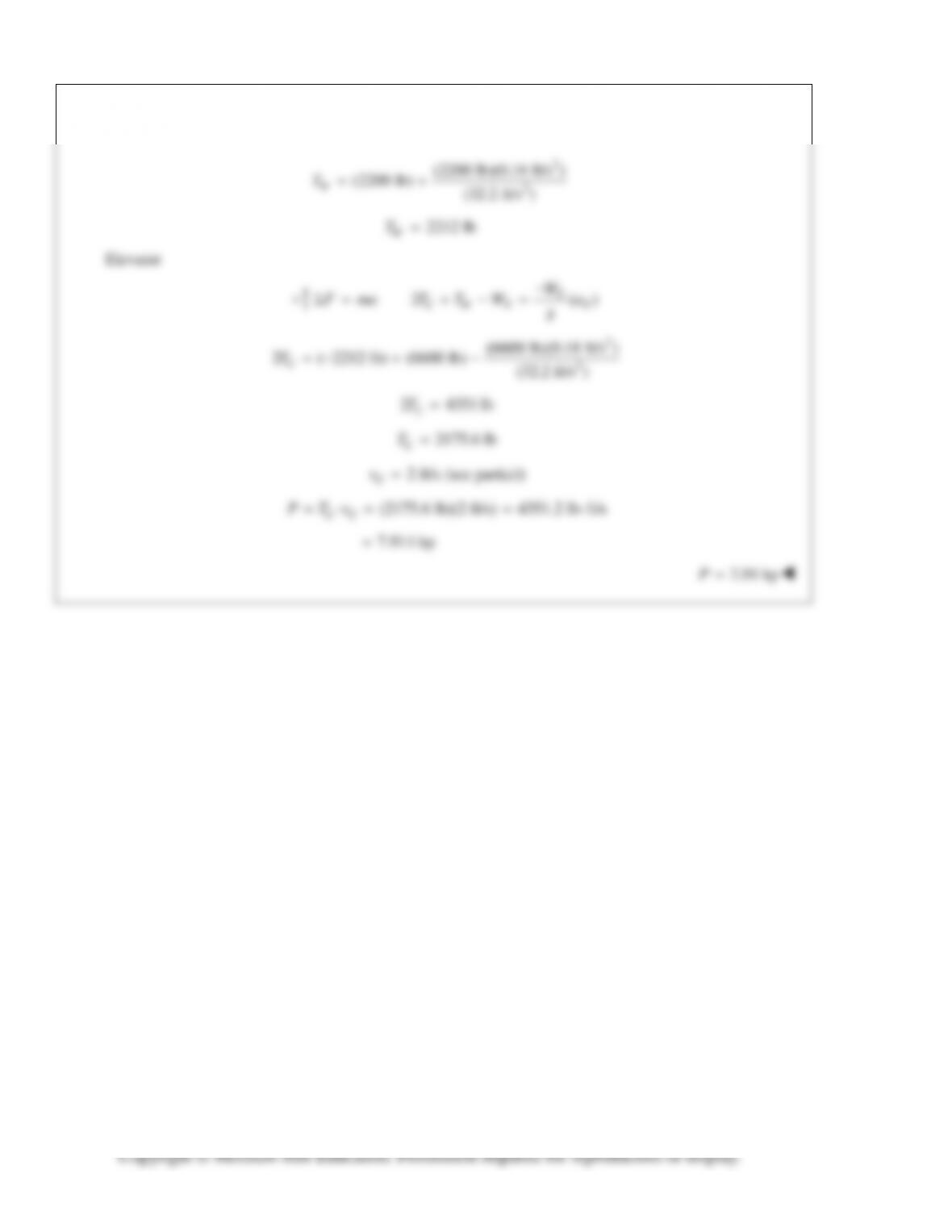PROBLEM 13.54 (Continued)